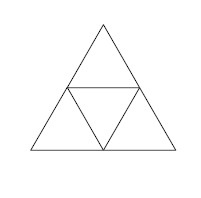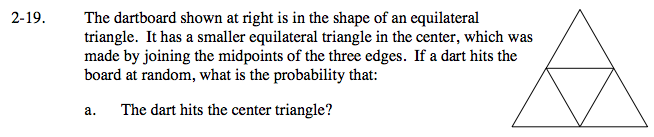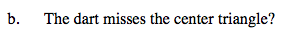Home > A2C > Chapter 2 > Lesson 2.1.1 > Problem2-19

2-19.
1. The dartboard shown below is in the shape of an equilateral triangle. It has a smaller equilateral triangle in the center, which was made by joining the midpoints of the three edges. If a dart hits the board at random, what is the probability that: Homework Help ✎1. The dart hits the center triangle?

2. The dart misses the center triangle?How many smaller triangles make up the bigger triangle?

$\text{The probability is }\frac{1}{4}.$How many smaller triangles can be hit if the center one is missed?